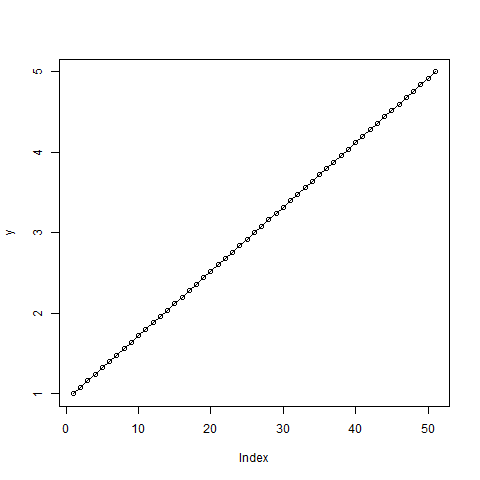# Compute the Value of Quantile Function over Uniform Distribution in R Programming – qunif() Function

In R programming, `qunif()` function gives the value of quantile function over Uniform Distribution.

Syntax:
qunif(p, min, max, lower.tail = TRUE, log.p = FALSE)

Parameters:
p: represents probabilities vector
min, max: represents lower and upper limits of the distribution
lower.tail: represents logical value. If TRUE, probabilities are P[Xx]
log.p: represents logical value. If TRUE, probabilities are given as log(p)

Example 1:

 `# R program to find the value of ` `# Quantile Function ` ` `  `# Creating a vector ` `x <- ``seq``(0, 1, by = 0.2) ` ` `  `# qunif() function ` `qunif``(x, min = 2, max = 6) `

Output:

``` 2.0 2.8 3.6 4.4 5.2 6.0
```

Example 2:

 `# Create variables ` `x <- ``seq``(0, 1, by = 0.02) ` `y <- ``qunif``(x, min = 1, max = 5) ` ` `  `# Output to be present as PNG file ` `png``(file = ``"qunifGFG.png"``) ` ` `  `# Plot ` `plot``(y, type = ``"o"``)  ` ` `  `# Saving the file ` `dev.off``() `

Output:My Personal Notes arrow_drop_upBlockchain Enthusiast

If you like GeeksforGeeks and would like to contribute, you can also write an article using contribute.geeksforgeeks.org or mail your article to contribute@geeksforgeeks.org. See your article appearing on the GeeksforGeeks main page and help other Geeks.

Please Improve this article if you find anything incorrect by clicking on the "Improve Article" button below.

Article Tags :

Be the First to upvote.

Please write to us at contribute@geeksforgeeks.org to report any issue with the above content.